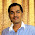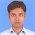Saturday, September 15, 2012

Explain with example traveling salesman problem?

The "Traveling Salesman Problem" (TSP) is a common problem applied to artificial intelligence. The TSP presents the computer with a number of cities, and the computer must compute the optimal path between the cities. This applet uses a genetic algorithm to produce a solution to the "Traveling Salesman Problem".

The traveling salesman problem (TSP) is a problem in discrete or combinatorial optimization. It is a prominent illustration of a class of problems in computational complexity theory, which are classified as NP-hard. In the traveling-salesman problem, which is closely related to the Hamiltonian cycle problem, a salesman must visit n cities. Modeling the problem as a complete graph with n vertices, we can say that the salesman wishes to make a tour, or hamiltonian cycle, visiting each city exactly once and to finishing at the city he starts from. There is an integer cost c(i, j) to travel from city i to city j , and the salesman wishes to make the tour whose total cost is minimum, where the total cost is the sum of the individual costs along the edges of the tour.

Representing the cities by vertices and the roads between them by edges. We get a graph. In this graph, with every edge there is associated a real number such a graph is called a weighted graph being the weight of edge.

In our problem, if each of the cities has a road to every other city, we have a complete weighted graph. This graph has numerators Hamiltonian circuits, and we are to pick the one that has the smallest sum of the distance

The total number of different Hamiltonian circuits in a complete graph of n vertices can be shown to be (n - 1)!/2. This follows from the fact that starting from any vertex we have n - 1 edges to choose from the first vertex , n- 2 from the second, n- 3 from the third, and so on, these being independent, result with (n-1) choices This number is, however, divided y2, because each Hamiftonian circuit has been counted twice
Theoretically the problem of the traveling salesman can always be solved by enumeration all (n-1)!/2 Hamtltonian circuits, calculation the distance traveled in each, and then picking the shortest one. However for a large value of n, the labor involved is too great even for a digital computer.
The problem is to prescribe a manageable algorithm for finding the shortest route. No efficient algorithm for problems of arbitrary size has yet been found, although many attempts have been made. Since this problem has application in operations research, some specific large-scale examples have been worked out. There are also available several heuristic methods of solution that give a route very close to the shortest one, but do not guarantee the shortest.

1.2.